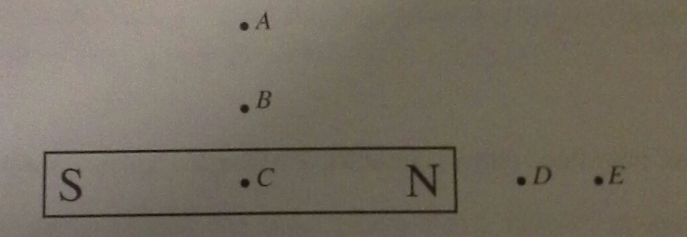# Problem: a) Carefully draw the magnetic field lines for the bar magnet shown below. Be sure to draw the field lines so that they include information about the strength and direction of the field both inside and outside the magnet.b) Based on the magnetic field you have drawn, rank the magnitude of the magnetic field at points A-E.

###### FREE Expert Solution

Magnetic field:

$\overline{){\mathbf{B}}{\mathbf{=}}\frac{{\mathbf{\mu }}_{\mathbf{0}}\mathbf{i}}{\mathbf{2}\mathbf{\pi }\mathbf{r}}}$

a)

The magnetic field lines come from the North pole to the South pole.

The magnetic field lines are also stronger towards the poles, implying that the field lines are denser at the poles.

86% (455 ratings)###### Problem Details

a) Carefully draw the magnetic field lines for the bar magnet shown below. Be sure to draw the field lines so that they include information about the strength and direction of the field both inside and outside the magnet.b) Based on the magnetic field you have drawn, rank the magnitude of the magnetic field at points A-E.